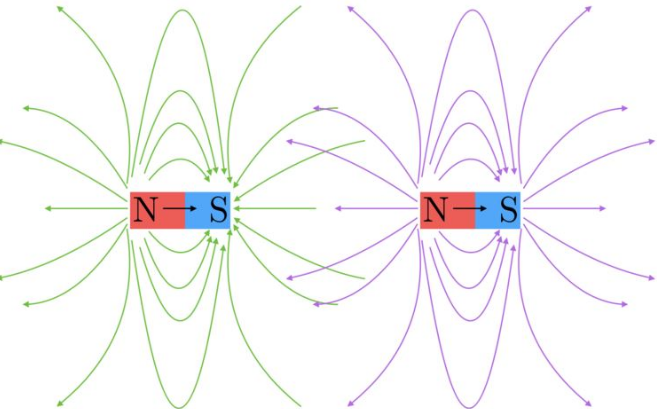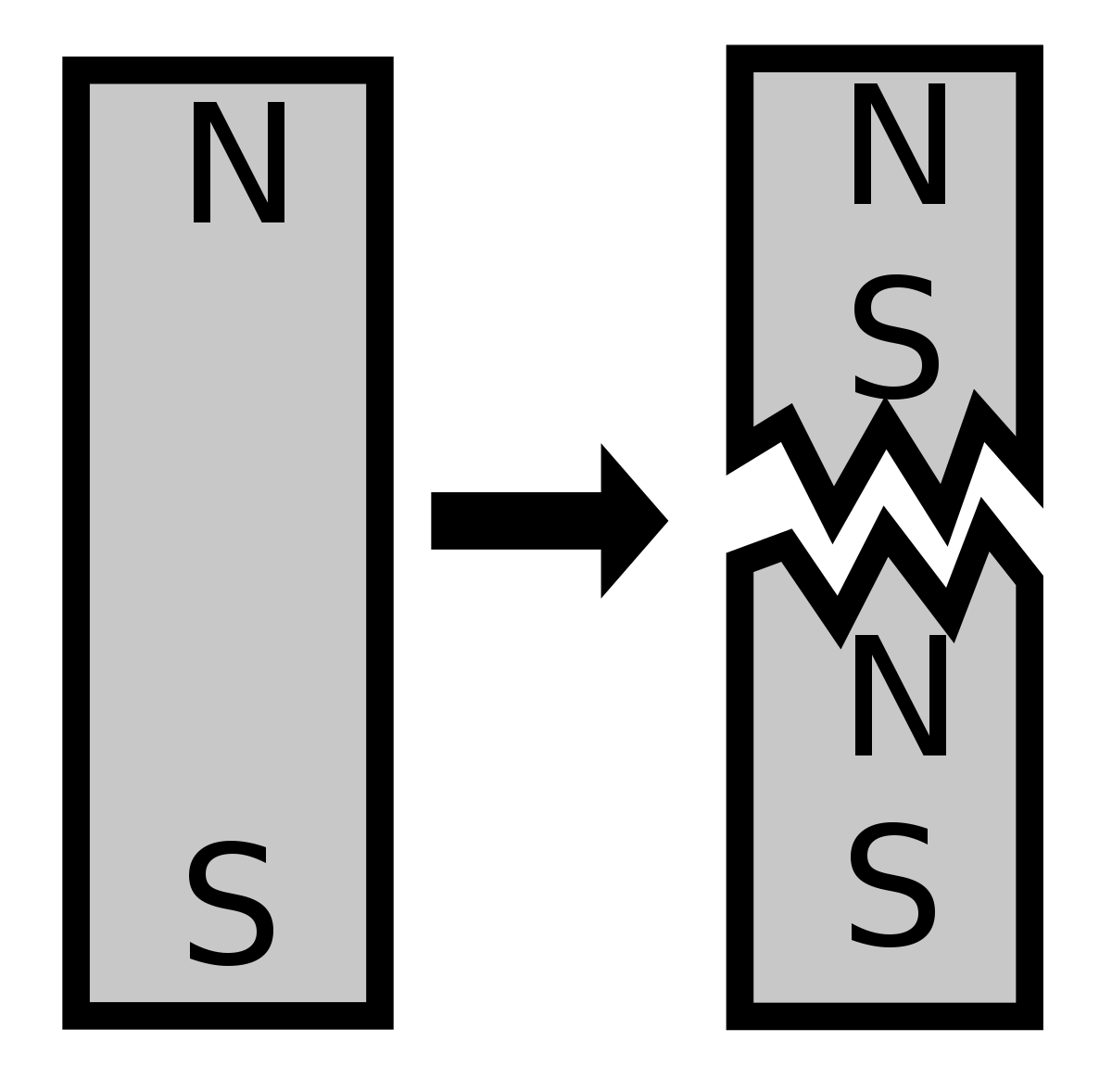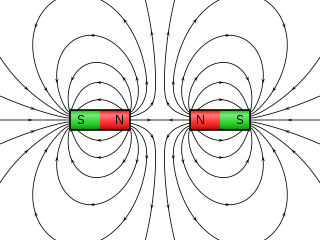# Magnetic Forces and Fields

• Astrophysics• Electricity• Electromagnetism• Energy• Fields• Force• Mechanics• Momentum• Nuclear Physics• Quantities & Units• States of Matter• Waves• Key ExperimentsHave you ever thought about how your microwave oven works? It's actually pretty cool - it uses the same principles as magnets! And did you know that if you have a piercing and go for an MRI scan, the piercing could get ripped off? Ouch! All of these things are caused by magnetic forces and fields. These fields can be teeny tiny, like the space around your fridge magnet, or as big as the earth.

To understand magnetic forces, let's first talk about what a force is. A force is something that happens when two objects interact and causes a change in their movement. It's like when you push or pull something.

So, magnets can push or pull each other and even charged particles. This is the basis for how the microwave oven was invented. Pretty cool, right? Let's find out more!

And that's how magnetic forces and fields make our lives more interesting and convenient, from heating up our food to keeping our notes on the fridge.

## Magnetic force and magnetic field definitions

When we talk about magnets, we need to understand the difference between a magnetic field and a magnetic force. Let's start with the magnetic force.

The magnetic force is what a charged particle (like an electron or proton) feels when it moves through a magnetic field. It's a force that's generated by magnets and measured in Newtons, just like any other force. But here's the catch - the charged particle has to be moving in relation to the magnetic field to feel this magnetic force.

Now, let's talk about the magnetic field. It's the area around a magnet where the magnetic force can be felt. So, any charged particle that enters this area will feel the magnetic force.

So, to sum up - a magnetic force is what a charged particle feels when it moves through a magnetic field, and a magnetic field is the area around a magnet where this force can be felt.

## Relationship between magnetic force and magnetic field

The magnetic field is the region in space where a moving charge or permanent magnet experiences a force. This force is known as the magnetic force and is a consequence of the electromagnetic force, one of the four fundamental forces of nature. The magnetic force is caused by the motion of charges and is typically calculated as a cross product. The unit of measurement of the magnetic field is the Tesla (T) which is equivalent to Newtons per Ampere per metre.

## Magnetic Poles

Magnetic objects have two poles - north (N) and south (S) - that determine whether they will attract or repel other poles. When we bring two bar magnets close to each other, we feel the magnetic forces between them. The magnetic forces are determined by the rule that like poles repel and unlike poles attract.

The magnetic force is present within the magnetic field of the magnet. So, when one magnetic pole feels a magnetic force, it's because it's within the magnetic field of another magnet. This means that magnetic poles not only produce magnetic fields, but they also get affected by the magnetic fields of other magnetic poles.

In summary, the interaction between bar magnets is due to the magnetic force that exists between their north and south poles. When brought close together, like poles repel and unlike poles attract. These interactions take place within the magnetic fields of the magnets.

## Magnetic field lines

Magnetic field lines are a way to represent the lines along which magnetic forces act. They are not visible, but rather mathematical abstractions that contain information about how the magnetic field affects moving charges in its vicinity at every point in space. We can infer their presence by the force experienced by magnetic objects placed at each point in the field, but it's the force that we measure, not the magnetic field strength itself.

The magnetic field lines are defined such that they always form closed loops, and the direction of the lines indicates the direction of the magnetic force acting on a north pole. The magnetic field lines also indicate the strength of the magnetic field at any point in space. The closer the lines are together, the stronger the magnetic field is at that point.

Magnetic field lines are a useful tool for describing the behavior of magnetic fields and are widely used in physics and engineering. They help us understand the interactions between magnetic objects and can be used to design devices such as motors, generators, and transformers.

In summary, magnetic field lines are a way to represent the lines along which magnetic forces act. They are not visible but are mathematical abstractions that contain information about the magnetic field's strength and direction. Magnetic field lines form closed loops, and their spacing represents the magnetic field's strength at different points in space.The magnetic field lines of a typical bar magnet indicated in this image tell us the direction of the force that would be exerted on a second north pole that enters this magnetic field

The magnetic field lines of a magnet start at the north pole and terminate at the south pole. The arrows on the field lines indicate the direction in which a north pole of a second magnet would feel a magnetic force if it enters the magnetic field of the first magnet. Like poles repel, so a north pole will be repelled by another north pole, and it will be attracted to a south pole.

When we cut a bar magnet at the center that separates the north and south magnetic poles, we get two smaller magnets, each with its own north and south poles. The resulting magnets will have weaker magnetic fields than the original magnet, but they will still have the same characteristics of attracting or repelling other magnets based on their polarity.

It is important to note that magnetic objects always exist as dipoles, meaning that they have both north and south poles. Even if we cut a magnet into smaller pieces, each piece will still have both poles. This is because the magnetic field is a fundamental property of the material, and it cannot be separated into individual parts.

In summary, cutting a bar magnet at the center will result in two smaller magnets, each with its own north and south poles. Magnetic objects always exist as dipoles, and even if we cut a magnet into smaller pieces, each piece will still have both poles.A magnet that is cut into smaller pieces will form smaller magnets each with its own north and south poles

## Examples of magnetic field and force

When two magnets are brought together in different orientations, the magnetic field lines between them can have different patterns. Let's take the example of bringing the north pole of one bar magnet near the south pole of another bar magnet, with their end faces parallel.

In this case, the magnetic field lines between the two unlike poles can be represented by starting at the north poles and ending at the closer of the two south poles. The magnetic field lines will curve around the magnets and will be denser where the magnets are closer together. The arrow direction on the field lines shows the direction in which a north pole of a second magnet would feel a magnetic force if it enters the magnetic field of the first.

The magnetic force between the two magnets is attractive, and the two magnets will move towards each other as long as there are no other forces, such as friction. This is because the magnetic field lines between the unlike poles of the two magnets are pointing towards each other, indicating an attractive force.

In summary, when the north pole of one bar magnet is brought near the south pole of another bar magnet with their end faces parallel, the magnetic field lines between the unlike poles will start at the north poles and end at the closer of the two south poles. The magnetic force between the two magnets is attractive, and the two magnets will move towards each other.The magnets attract each other and the direction of the field lines are different at different points

## The formula relating magnetic force and magnetic field

F = BIL

where F is the force experienced by the wire, B is the magnitude of the magnetic field, I is the current flowing through the wire, and L is the length of the wire that is perpendicular to the magnetic field.

This equation shows that the force experienced by the wire is directly proportional to the magnetic field strength, the current flowing through the wire, and the length of the wire that is perpendicular to the magnetic field. This means that the greater the magnetic field strength or the current flowing through the wire, the greater the force experienced by the wire. Similarly, the longer the wire that is perpendicular to the magnetic field, the greater the force experienced by the wire.

This phenomenon is known as the Lorentz force and is a fundamental concept in electromagnetism. The Lorentz force explains how electric currents can interact with magnetic fields, and it is the basis for many practical applications, such as electric motors and generators.

In summary, a wire carrying an electric current will experience a force when placed in a uniform magnetic field. The force experienced by the wire is given by the Lorentz force equation, which is directly proportional to the magnetic field strength, the current flowing through the wire, and the length of the wire that is perpendicular to the magnetic field. The Lorentz force is a fundamental concept in electromagnetism and has many practical applications.

## Magnetic Forces and Fields

What is magnetic field force?

It is the force felt by a charged particle (electron, proton, ion, etc.) when it enters a magnetic field.

What is the relationship between force and magnetic field?

A magnetic field is a region in space where a moving charge or permanent magnet feels a force.

Are magnetic fields force fields?

Yes, it applies a magnetic force over a region of space and so it is a force field.

What is an example of magnetic force and field?

When the poles of two magnets are brought together, there will be an attractive force if the poles are opposite (i.e. north pole meets south pole) and an attractive force if unlike poles meet.

What is the formula for finding magnetic force and field?

For a particle with charge q moving with speed v at right angles to the magnetic field lines. The relationship between the magnetic force and field B is given by14-day free trial. Cancel anytime.Join 10,000+ learners worldwide.The first 14 days are on us96% of learners report x2 faster learningFree hands-on onboarding & supportCancel Anytime